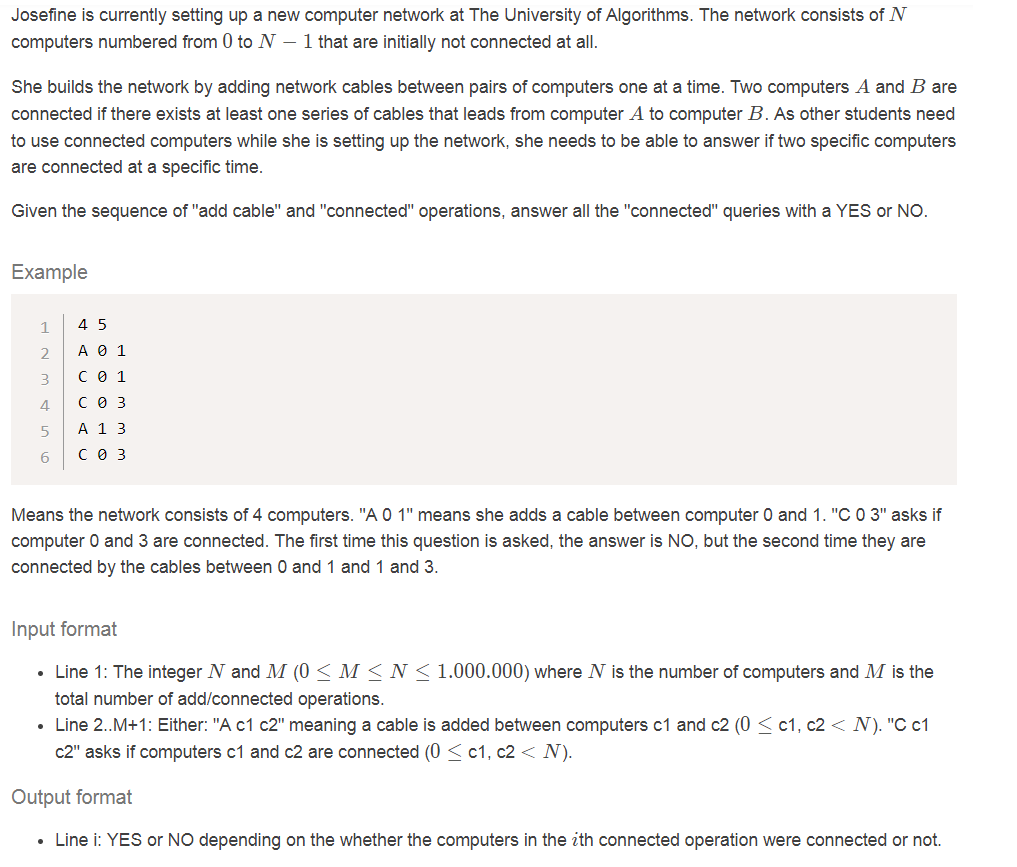# Hey I need help with a Java Program, when it is compiled it will be focusing on running time as well.

QuestionsCategory: Computer ScienceHey I need help with a Java Program, when it is compiled it will be focusing on running time as well.

Hey I need help with a Java Program, when it is compiled it will be focusing on running time as well.
Is it possible to make a program in Java that can solve the task, and make it run fast?FIRST TEST
INPUT (stdin)
4 5
A 0 1
C 0 3
C 0 1
A 1 3
C 0 3
Expected Output
NO
YES
YES
SECOND TEST
INPUT (stdin)
10 20
A 5 1
C 7 5
C 7 2
C 5 3
A 2 5
C 8 5
A 1 0
A 8 5
C 7 1
A 6 5
C 0 3
Expected Output
NO
NO
NO
NO
NO
NO
YES
NO
Hope you can help 🙂

import java.util.ArrayList;
import java.util.Scanner;
import java.util.stream.Collectors;
public class ConnectionOperations {

public static void main(String[] args)
{
Scanner sc = new Scanner(System.in);
ArrayList<Integer> connectedComputers = new ArrayList<>();
ArrayList<String> yesNo = new ArrayList<>();

System.out.print(“Enter number of computers (N) and number of connection operations (M) separated by spaces: “);
int N = 0;
int M = 0;
String operation = “”;
int c1 = 0, c2 = 0;

do
{

//System.out.println(“Values of N and M should be between 0 and 1000000.”);
//System.out.print(“Enter number of computers (N) and number of connection operations (M) separated by spaces: “);
N = sc.nextInt();
M = sc.nextInt();
}while((N < 0 || N > 1000000) && (M < 0 || M > 1000000));

sc.nextLine();

System.out.println(“Enter the operations: “);
for(int i = 0; i < M; i++)
{
operation = sc.nextLine().toUpperCase();
if(operation.charAt(0) == ‘A’)
{
String s = “”;
c1 = Integer.parseInt(operation.substring(operation.indexOf(” “) + 1, operation.lastIndexOf(” “)));
c2 = Integer.parseInt(operation.substring(operation.lastIndexOf(” “) + 1));
if(connectedComputers.contains(c1) && !connectedComputers.contains(c2))
{
if(c1 < c2)
{
for(int j = c1+1; j <= c2; j++)
{
}
}
else if(c2 < c1)
{
for(int j = c2+1; j < c1; j++)
{
}
}
}
else if(!connectedComputers.contains(c1) && connectedComputers.contains(c2))
{
if(c1 < c2)
{
for(int j = c1; j < c2; j++)
{
}
}
else if(c2 < c1)
{
for(int j = c2+1; j <= c1; j++)
{
}
}
}
else if(!connectedComputers.contains(c1) && !connectedComputers.contains(c2))
{
if(c1 < c2)
{
for(int j = c1; j <= c2; j++)
{
}
}
else if(c2 < c1)
{
for(int j = c2; j <= c1; j++)
{
}
}
}
}

if(operation.charAt(0) == ‘C’)
{
boolean found = false;
connectedComputers = (ArrayList)connectedComputers.stream().distinct().collect(Collectors.toList());
//String s = “”;
c1 = Integer.parseInt(operation.substring(operation.indexOf(” “) + 1, operation.lastIndexOf(” “)));
c2 = Integer.parseInt(operation.substring(operation.lastIndexOf(” “) + 1));

for(int conn : connectedComputers)
{
if(conn == c1)
{
found = true;
}
else
{
found = false;
}
}
for(int conn : connectedComputers)
{
if(conn == c2)
{
found = true;
}
else
{
found = false;
}
}
if(found)
{
//break;
}
else
{